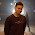### leetcode Question 103: String to Integer (atoi)

String to Integer (atoi)

Implement atoi to convert a string to an integer.
Hint: Carefully consider all possible input cases. If you want a challenge, please do not see below and ask yourself what are the possible input cases.
Notes: It is intended for this problem to be specified vaguely (ie, no given input specs). You are responsible to gather all the input requirements up front.

Analysis:
As there is no need to consider float number, what we need concern here is
(1) "+" and "-"
(2) The boundary INT_MAX and INT_MIN
(3) Eliminate the spaces before.
(4) Meet non-digit after digit then return.

### Code(Updated 201309):

class Solution {
public:
int atoi(const char *str) {
// Start typing your C/C++ solution below
// DO NOT write int main() function
if (!str){return 0;}
int i=0;
bool pos=true;
int res=0;
while (str[i]==' '){ i++;}
if (str[i]=='+'){ pos=true;i++;}
if (str[i]=='-'){ pos = false;i++;}
if (!isdigit(str[i])){return 0;}
while (isdigit(str[i])){
if (pos && res>INT_MAX/10){return INT_MAX;}
if (pos && res==INT_MAX/10 && int(str[i]-'0')>=7){return INT_MAX;}
if (!pos && -res<INT_MIN/10){return INT_MIN;}
if (!pos && -res==INT_MIN/10 && int(str[i]-'0')>=8){return INT_MIN;}
res = res*10 + int(str[i]-'0');
i++;
}

if (pos){return res;}
else{return -res;}

}
};


1.You need to change the "if (str[i]=='-')" to "else if (str[i]=='-')", otherwise it will give wrong ans on test cases like +-5 because at first str[i] == '+' will become true and will set pos to true and will increment i and then it will satisfy the second condition str[i] == '-' and will set pos to false and again increment the value of i. So finally the output will come as -5 which is wrong, because in this case the output should come as 0. changing the "if" condition to "else if" will serve the purpose.

1.I don't understand why the answer for "+-5" should be 0 and not -5
see this http://ideone.com/tBIC5b

2.Sumit is right. Your code does accept +-2.

3.The if of str[i]!='-' should be written in else if.